NOTE: This section is Under-Edit if necessary: Construction began on August 9, 2012 and finished on August 20, 2012.

Last Update: Addition to this section began on October 23, 2013 for the introduction of the Soft Decision Constellation Demapper (Bit Metrics application) for the Rate Matching Punctured Convolutional M-ary Signaling capability in AdvDCSM Professional.

This capability supplements the addition in February 26, 2013 for Rate Matching Convolutional and Punctured Convolutional Signaling capability and the addition in October 11, 2012 and finished on October 12, 2012 for the introduction of the Punctured Convolutional Codes capability in AdvDCSM Professional.

I) NON-SQUARE M-ARY QAM APPLICATION EXAMPLES

II) PUNCTURED CONVOLUTIONAL CODES (PCC) & APPLICATION EXAMPLES

III) RATE MATCHING (RM) APPLICATION EXAMPLES

IV) RM PCC SOFT DECISION CONSTELLATION DEMAPPER (BIT METRICS) APPLICATION EXAMPLES

V) SOFT-DECISION OUTPUTS DECODING (SOVA & 'Symbol-by-Symbol' MAP ALGORITHM)

VI) TURBO CODED SIGNALING over a COHERENT MEMORYLESS CHANNEL: BPSK SIGNALING and the ITERATIVE TURBO DECODER

Today and going forward into the future, there exist digital communications applications that require both high Spectral Efficiency and high Power Efficiency. Such applications involving wireless communications need to function in harsh band-limited environments and in mobile environments where power intensity is battery dependent.

Three design approaches for these wireless applications is to use High-Rate Convolutional Codes (Rate b/n) coupled with the Viterbi Decoding Algorithm, Non-Square M-ary Quadrature Amplitude Modulation (M-QAM) Modulation Schemes, and Rate Matching. To achieve a High-Rate Convolutional Code, the concept of puncturing a rate 1/n code is used so that the complexity of the Viterbi decoder is minimized. And by applying an appropriate Non-Square M-QAM constellation, a high-speed data transmission can occur while both peak and average power is reduced for a given impaired signaling channel. Further, in channels of varying Signal-to-Interference plus Noise Ratio, Rate Matching can be used to support Adaptive Modulation. Rate Matching provides the capability to create a modulation that is independent of the coding/puncturing structure.

AdvDCSM Professional can be used to investigate interesting problems and advanced applications that involve Non-Square (NS) M-QAM modulation schemes, or/and Punctured convolutional codes (PCC), or/and Rate Matching Unpunctured convolutional or Punctured convolutional codes in digital communication systems.

I) The AdvDCSMT1DCSS (T1) Version 1 system tool provides the capability to model and simulate Convolutional Coded, Block Coded or UnCoded M-ary Quadrature Amplitude Modulation (M-QAM) Signaling over Memoryless, Memory Channels, or Parallel MultiChannels with Additive White Gaussian Noise (AWGN) using SQUARE QAM (Even-Bit) constellations (4-QAM, 16-QAM, and 64-QAM).

There are advanced digital communications system applications such as high-speed wireless digital communications that require the use of NON-SQUARE M-ary QAM that consists of a Rectangular, Cross, or Non-Rectangular 2-D Signal Vector Space (constellation) for Signaling over complex bandwidth-limited and power-limited channels.

The following five journal and conference papers provide important background information about the NON-SQUARE M-ary QAM:

1) Joel G. Smith, "Odd-Bit Quadrature Amplitude-Shift Keying", IEEE Transactions on Communications, Vol. 23, No. 3, pp. 385-389, March 1975.

2) K. Cho, D. Yoon, W. Jeong, and M. Kavehrad, "BER Analysis of Arbitrary Rectangular QAM", Conference Record of the Thirty-Fifth Asilomar Conference on Signals, Systems and Computers, 2001., Vol. 2, pp. 1056-1059, Nov. 4-7, 2001.

3) P.K. Vitthaladevuni and M-S Aloluini,"Exact BER Computation for the Cross 32-QAM Constellation", First International Symposium on Control, Communications and Signal Processing, 2004., pp. 643-646, Sept. 27, 2004.

4) L. Li, D. Divsalar, and S. Dolinar, "Iterative Demodulation, and Decoding of Coded Non-Square QAM", IEEE Transactions on Communications, Vol. 53, No. 1, pp. 16-19, January 2005.

5) P.K. Vitthaladevuni and M-S Aloluini, "Exact BER Computation for Cross 32-QAM Constellations", IEEE Transactions on Wireless Communications, Vol.4, No. 6, pp. 3039-3050, November 2005.

T1 Version 2, the Professional Version of T1, has been developed that will provide to the User the capability/feature of using NON-SQUARE M-ary QAM, M = 8, 16, 32, and 64 in complex Digital Communication Systems (modeling and simulation).

Also, T1's Natural Language (NL) 'is' Input Method for Version 2 has been enhanced so that its Reliability of Input of Digital Communication System Model and Simulation Specifications will be increased.

Consider the Non-Square M-QAM Signaling example, Rectangular and Non-Rectangular 8-QAM, Bit Error Rate (BER) or bit error probability Pb performance simulation results that were produced by T1 Professional that are displayed below in Figure 1 and Figure 2 plots.

The Rectangular 8-QAM and the Non-Rectangular 8-QAM constellations that were used in the T1 simulations are displayed below in Figure 3 and Figure 4: Gray Coded Rectangular 8-QAM and Gray Coded Non-Rectangular 8-QAM, respectively.

From the T1 simulated BER results plot, one can observe easily the important benefit of the use of Convolutional Channel Coding to reduce the BER for a given 8-QAM Signaling Scheme and Information Bit Eb/No (& Average Channel Symbol Es/No).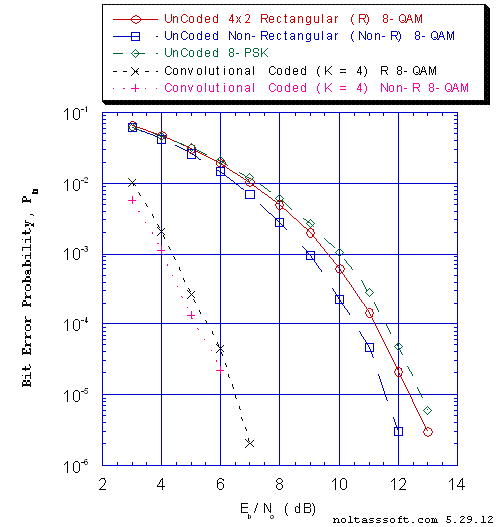Figure 1. Bit Error Probability for UnCoded 8-QAM Signaling, UnCoded 8-PSK
Signaling, and K = 5 Convolutional Coded 8-QAM Signaling over a Coherent
Memoryless Channel with Additive White Gaussian Noise (AWGN):
Rectangular [4x2 (IxQ)] 8-QAM signal constellation with Gray encoding;
Non-Rectangular 8-QAM signal constellation with 'Impure' Gray encoding;
8-PSK (a circular signal constellation scheme) with Gray encoding; &
Rate = 1/3, Constraint Length K = 4, (7, 5, 6, 7), a Best (Optimal)
Non-Recursive Convolutional Code (J.P. Odenwalder) and Viterbi Algorithm
Decoder using a Path Memory Length of 25 bits and an Unquantized Branch
Metric.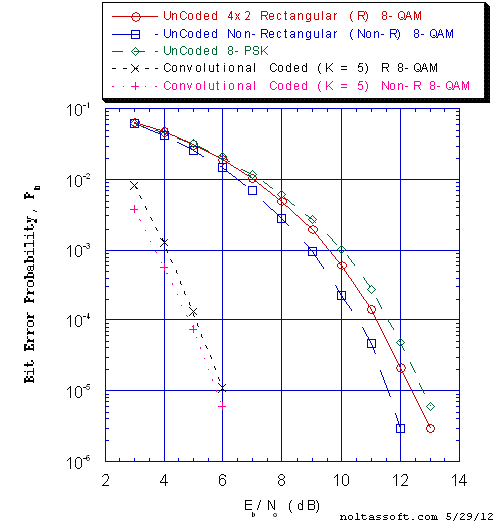Figure 2. Bit Error Probability for UnCoded 8-QAM Signaling, UnCoded 8-PSK
Signaling, and K = 5 Convolutional Coded 8-QAM Signaling over a Coherent
Memoryless Channel with Additive White Gaussian Noise (AWGN):
Rectangular [4x2 (IxQ)] 8-QAM signal constellation with Gray encoding;
Non-Rectangular 8-QAM signal constellation with 'Impure' Gray encoding;
8-PSK (a circular signal constellation scheme) with Gray encoding; &
Rate = 1/3, Constraint Length K = 5, (7, 6, 5, 6, 7), a Best (Optimal)
Non-Recursive Convolutional Code (J.P. Odenwalder) and Viterbi Algorithm
Decoder using a Path Memory Length of 25 bits and an Unquantized Branch
Metric.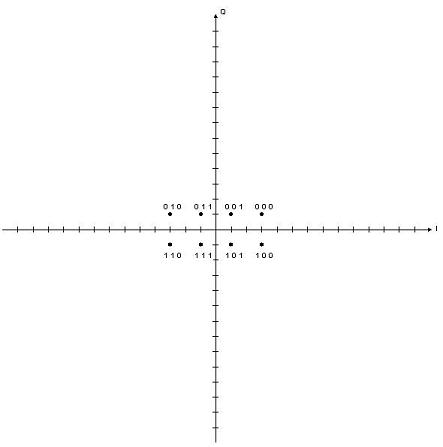Figure 3. Gray Coded Rectangular 8-QAM constellation.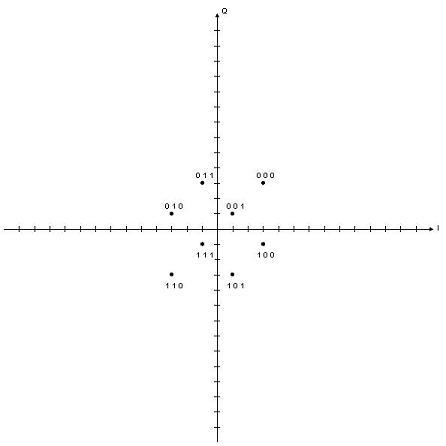Figure 4. Gray Coded Non-Rectangular 8-QAM constellation.

Next, Consider the Peak Channel Symbol Signal-to-Noise Ratio (SNR) Es/No results that correspond to the Convolutional Coded (K = 4) 8-QAM Signaling simulations that are displayed in the Figure 5 plot.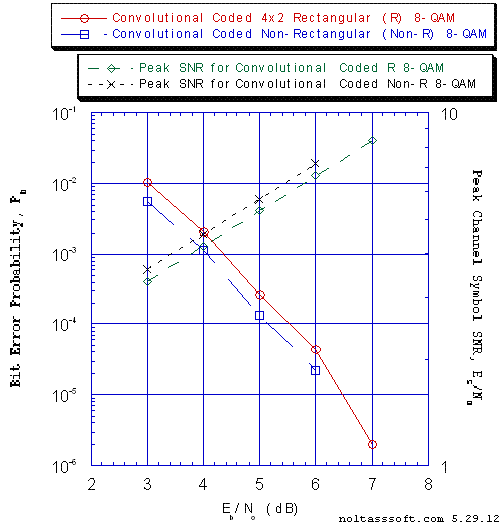Figure 5. Bit Error Probability and Peak Channel Symbol Signal-to-Noise Ratio (SNR),
Es/No for Convolutional Coded 8-QAM Signaling over a Coherent Memoryless
Channel with Additive White Gaussian Noise (AWGN):
r = 1/3, K = 4, (7, 5, 6, 7) a Best (Optimal) Non-Recursive Convolutional
Code (J.P. Odenwalder) and Viterbi Algorithm Decoder using a Path Memory
Length of 25 bits and an Unquantized Branch Metric;
Rectangular [4x2 (IxQ)] 8-QAM signal constellation with Gray encoding; &
Non-Rectangular 8-QAM signal constellation with 'Impure' Gray encoding.

There are many important conclusions that can be drawn from the above displayed NON-SQUARE 8-QAM (Odd-Bit) Signaling BER and Peak Channel SNR results that were produced by T1 Professional.

But here the focus is on the operation of T1 Professional: it appears that T1 is correctly modeling and simulating NON-SQUARE M-ary QAM (Odd-Bit). And NON-SQUARE 64-QAM Signaling as well as NON-SQUARE 16-QAM, or NON-SQUARE 32-QAM (Odd-Bit) can be modeled and simulated easily in T1 Professional, too.

So now T1 in its Professional version can model and simulate correctly both NON-SQUARE and SQUARE M-ary QAM.

Further, T1 Professional allows the User to easily construct each NON-SQUARE constellation using a new Custom M-ary QAM Constellation Visual Builder/Mapper and a Custom M-ary QAM Bit-to M-ary QAM Channel Symbol Converter (Non-Square) Visual Builder/Mapper.

And upon creation of a NON-SQUARE constellation and a Bit-Symbol Mapping (using the M-ary Modulation Scheme Validation/Design/Edit Design feature via the Design Menu), a User can save the particular MQAM Scheme parameters in a data file. This MQAM Scheme Parameters Data file can then be used for specifying a M-ary QAM Scheme's parameters at time of input for a Coded or UnCoded M-ary QAM Signaling over a Memoryless or Memory Single Channel Simulation.

This feature allows the User to construct a very complex Non-Square MQAM Constellation (& associated Bit-Symbol Mapping) one time and use it for many simulations. Also, the User can edit this constellation (& Bit-Symbol Mapping) and save the new constellation (& Bit-Symbol Mapping) in another data file.

II) The AdvDCSMT1DCSS (T1) Professional system tool provides the capability to model and simulate Punctured Convolutional Coded (PCC) Signaling over a Memoryless Channels (MLC) with Additive White Gaussian Noise (AWGN). The capability to puncture a b = 1 convolutional code and to use that punctured code to signal over a Noiseless, BSC, or AWGN MLC has been incorporated into the development version of T1 Professional (T1 Version 2).

Consider the first PCC Binary Phase-Shift Keying (BPSK) Bit Error Rate (BER) or Bit Error Probability Pb performance simulation results that were produced by T1 that are displayed below in the Figure 6, 7, and 8 plots.

The parent code used for puncturing to achieve the high-rate (n - 1) / n codes for Rates = {2/3, 3/4, 4/5, 5/6, 6/7, 7/8, or 8/9} is a Rate = 1/2 Constraint length K = 5 Odenwalder code [a Best (Optimal) Non-Recursive convolutional code].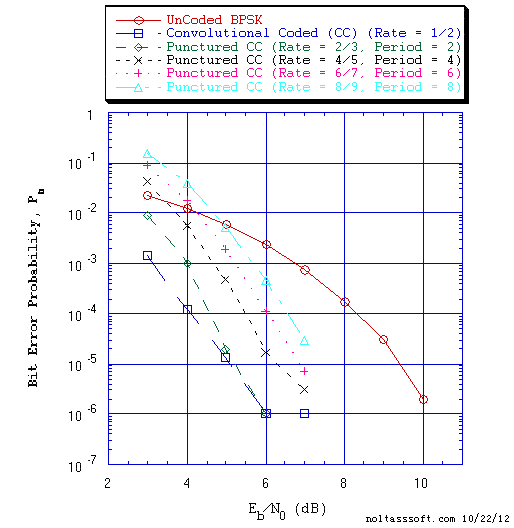Figure 6. Bit Error Probability for UnCoded BPSK Signaling, Convolutional Coded
BPSK Signaling, and Punctured Convolutional Coded BPSK Signaling over a
Coherent Memoryless Channel with Additive White Gaussian Noise (AWGN):
Parent Code Rate = 1/2, K = 5, (3, 1, 2, 2, 3), a Best (Optimal)
Non-Recursive Convolutional Code (J.P. Odenwalder) and Viterbi Algorithm
Decoder using a Path Memory Length of 60 bits and an Unquantized Branch
Metric;
Punctured Codes derived from this 1/2 rate parent code for Even-Number
Puncturing Period (P = {2,4,6,8}):
Punctured Rate = 2/3, P Matrix [[1,0], [1,1]];
Punctured Rate = 4/5, P Matrix [[1,1,0,1], [1,0,1,0]];
Punctured Rate = 6/7, P Matrix [[1,1,0,1,0,1], [1,0,1,0,1,0]]; &
Punctured Rate = 8/9, P Matrix [[1,1,0,1,1,1,0,0], [1,0,1,0,0,0,1,1]].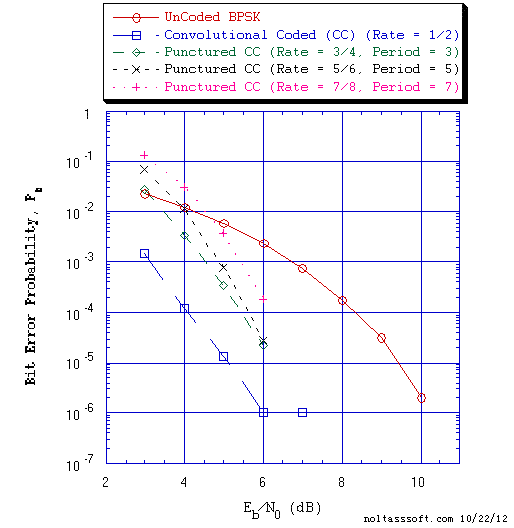Figure 7. Bit Error Probability for UnCoded BPSK Signaling, Convolutional Coded
BPSK Signaling, and Punctured Convolutional Coded BPSK Signaling over a
Coherent Memoryless Channel with Additive White Gaussian Noise (AWGN):
Parent Code Rate = 1/2, K = 5, (3, 1, 2, 2, 3), a Best (Optimal)
Non-Recursive Convolutional Code (J.P. Odenwalder) and Viterbi Algorithm
Decoder using a Path Memory Length of 60 bits and an Unquantized Branch
Metric;
Punctured Codes derived from this 1/2 rate parent code for Odd-Number
Puncturing Period (P = {3,5,7}):
Punctured Rate = 3/4, P Matrix [[1,1,0], [1,0,1]];
Punctured Rate = 5/6, P Matrix [[1,1,0,0,0], [1,0,1,1,1]]; &
Punctured Rate = 7/8, P Matrix [[1,1,0,1,1,0,0], [1,0,1,0,0,1,1]].
The 1979 paper by J. Bibb Cain et al. (PCC Reference No. 1) and 1984 paper by Y. Yasuda et al. (PCC Reference No. 2) provide valuable insight into the problem of puncturing a b = 1 convolutional code in an attempt to reduce the complexity of the Viterbi Algorithm (VA) Decoder as applied to decoding high rate codes.

For each Punctured Code case, the P Matrix was derived from the í column (í, Forney's definition of constraint length) in Table I on page 317 of the Yasuda et al. paper. Using Forney's definition of constraint length (number of memory units), í = 4 corresponds to T1's constraint length of K = 5.

It is important to realize that the rule of Path Memory Length (PML) of 5bK for optimal decoding of Convolutional Codes is not applicable to the VA decoding of Punctured Convolutional Codes. This PML choice for a particular punctured code and its parent code K is a complex matter. For this work, the 12bK PML or 60 bits was chosen.

It is interesting that Cain et al. and Yasuda et al. papers do not display any simulated Punctured Codes BER Performance results. The Cain et al. paper shows Union bounding technique-base BER performance results for punctured rates R = 2/3 (Fig. 3 plot) and R = 3/4 (Fig. 4 plot) on page 100. Note that Cain's paper does claim agreement with simulation results (page 100). In the Yasuda et al. paper, Fig. 2. BER performance of rate 7/8 punctured codes results plot (page 318) is shown but it is stated that these results are calculated (page 316).

Next, simulated BER results produced by T1 for a Rate-Compatible Punctured Convolutional (RCPC) code are shown below in the Figure 8 plot. The parent code used for puncturing to achieve the RCPC rate = 4/9 is a Rate = 1/3 Constraint length K = 4 Odenwalder code [a Best (Optimal) Non-Recursive convolutional code]. The Puncturing Matrix P is derived from Table 8.4-2 on page 524 of Digital Communications (J.G. Proakis et al., PCC Reference 3).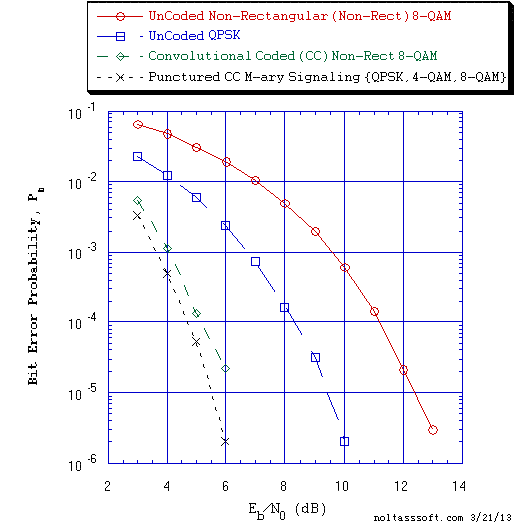Figure 8. Bit Error Probability for UnCoded 8-QAM Signaling, UnCoded QPSK Signaling,
Convolutional Coded 8-QAM Signaling, and Punctured Convolutional Coded
M-ary Signaling over a Coherent Memoryless Channel with Additive White
Gaussian Noise (AWGN):
Parent Code Rate = 1/3, K = 4, (7, 5, 6, 7) a Best (Optimal) Non-Recursive
Convolutional Code (J.P. Odenwalder) and Viterbi Algorithm Decoder using a
Path Memory Length of 48 bits and an Unquantized Branch Metric;
Rate = 4/9 Punctured Code derived from this 1/3 rate parent code for
Puncturing Period P = 8 using the following P Matrix:
[[1,1,1,1,1,1,1,1], [1,1,1,1,1,1,1,1], [1,0,0,0,1,0,0,0]];
M-ary Signaling Schemes: {Non-Rect 8-QAM, QPSK, Square 4-QAM, QPSK,
Non-Rect 8-QAM, QPSK, Square 4-QAM, QPSK}; &
Non-Rect 8-QAM: 'Impure' Gray Coded; QPSK & 4-QAM: Gray Coded.
Note that the T1 rate 4/9 RCPC coded signaling over a MLC simulations used M-QAM scheme modulated signals that were taken from both Square and Non-Square (Non-Rectangular) constellations (Signal Vector Spaces). The 8-QAM Non-Rectangular signal vector locations (constellation) match the non-square 8-QAM constellation locations in a 2002 IEEE 802.16 Broadband Wireless Access Work Group proposal (PCC Reference 4) and its shape is displayed in Figure 4 above.

There are many important conclusions that can be drawn from the above displayed PCC MLC Signaling results for BPSK and M-ary linear modulation (PSK, QAM) schemes. But here the focus is on the operation of T1: it appears that T1 is correctly modeling and simulating Punctured Convolutional Coded Signaling over an AWGN Memoryless Channel. And other parent codes with rates of 1/2, 1/3, 1/4, 1/5, 1/6 codes for Puncturing Periods of P = 2, 3, 4, 5, 6, 7, or 8 can be modeled and simulated easily, too. Also, the choice of the first code bit of branches 2 through P of a Puncturing Period being deleted is included in T1. Further, T1 allows the User to easily construct each Punctured Convolutional code using a new Punctured Convolutional Code Visual Builder and Punctured Convolutional Coded Multiple Channel Modulations Visual Builders for Linear Modulation (PSK, PAM, QAM) or Non-Linear Modulation Schemes (FSK) and their corresponding Received or Transmitted Signal-to-Noise Ratio (SNR)s.

Punctured Convolutional Code (PCC) References:

1) J. Bibb Cain, George C. Clark, Jr., and John M. Geist, "Punctured Convolutional Codes of Rate (n-1) / n and Simplified Maximum Likelihood Decoding, IEEE Transactions on Information Theory, Vol. IT-25, No. 1, pp. 97-100, January 1979.

2) Yutaka Yasuda, Kanshiro Kashiki, and Yasuo Hirata, "High-Rate Convolutional Codes for Soft Decision Viterbi Decoding", IEEE Transactions on Communications, Vol. COM-32, No. 3, pp. 315-319, March 1984.

3) John G. Proakis and Masoud Salehi, Digital Communications, Fifth Edition, McGraw-Hill, Boston, 2008.

4) J.A. Torres, "Method for using non-squared QAM constellations", IEEE 802.16 Broadband Wireless Access Working Group, http://www.ieee802.org/16/tga/contrib/C80216a-02_66.pdf, May 2002.

III) The AdvDCSMT1DCSS (T1) Professional system tool provides the capability to model and simulate Rate Matching (RM) Convolutional Coded (CC) or RM Punctured Convolutional Coded (PCC) Signaling over Memoryless Channels (MLC) with Additive White Gaussian Noise (AWGN). Also, this capability of T1 Professional (T1 Version 2) supports simulated Rate Matching Signaling over a Noiseless or BSC MLC, too.

Consider the first Rate Matching PCC Bit Error Rate (BER) or Bit Error Probability Pb performance simulation results that were produced by T1 that are displayed below in Figure 9 plot.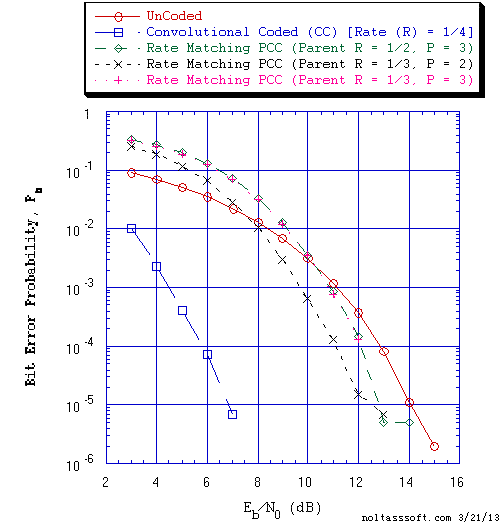Figure 9. Bit Error Probability for UnCoded, Convolutional Coded, and Rate Matching
Punctured Convolutional Coded (PCC) Non-Rectangular 16-QAM Signaling over
a Coherent Memoryless Channel with Additive White Gaussian Noise (AWGN):
Rate = 1/4, Constraint Length K = 4, (15,9,7,15), Parent Code Rates = 1/2,
K = 4, (3,2,3,3), & 1/3, K = 4, (7,5,6,7) Best (Optimal) Non-Recursive
Convolutional Codes and Viterbi Algorithm Decoder using a Path Memory
Length of 48 bits;
PCC Rate = 3/4 code derived from the 1/2 rate parent code for Puncturing
Period P = 3 using a P Matrix [[1,0,0],[1,1,1]];
PCC Rate = 2/4 code derived from the 1/3 rate parent code for P = 2 using
a P Matrix [[1,0],[1,1],[0,1]]; &
PCC Rate = 3/4 code derived from the 1/3 rate parent code for P = 3 using
a P Matrix [[1,0,0],[1,1,0],[0,0,1]].
Note that the 16-QAM Non-Rectangular signal vector locations (constellation) are based on a modified square constellation such that each corner vector is moved diagonally by a counterclockwise rotation to the first available location next to the I or Q axis. And each signal vector's Bit-to-M-ary Channel Input Symbol assignment is based upon a Gray Code scheme.

Further, it is important to realize that one 16-QAM channel symbol is transmitted and received per a trellis branch for the Non-Rate Matching Convolutional Coded (CC) signaling cases. And for the Rate Matching (RM) Punctured Convolutional (PCC) signaling cases, one 16-QAM channel symbol is transmitted and received per a Punctured Period of trellis branches.

One must note that the Viterbi Algorithm (VA) decoding process for Rate Matching PCC Signaling that uses Soft Decision Demodulation per Puncturing Period (a multiple of trellis branches) and a Hamming distance Branch Metric per trellis branch is more complicated than the VA decoding process for Non-Rate Matching CC Signaling that uses a Soft Decision Unquantized Branch Metric per trellis branch.

From the T1 simulated BER results plot, one can observe the complex behavior exhibited by the application of RM Punctured Convolutional Channel Coding. Clearly, the Non-Rate Matching CC 16-QAM Signaling BER Performance is must better than the Rate Matching PCC 16-QAM Signaling BER performance at low Information Bit Signal-to-Noise Ratio Eb/N0 (& Average Channel Symbol Signal-to-Noise Ratio Es/N0). But depending on the Eb/N0 value, the RM PCC BER performance is worst or better than the UnCoded 16-QAM BER performance. Note at a high Eb/N0 value and equivalent Bit Error Probability Pb the information bit throughput for PCC signaling is three (Rp = 3/4) or two (Rp = 2/4) times higher than the information bit throughput for CC signaling (R = 1/4).

There are some important conclusions that can be drawn from the above displayed Rate Matching Punctured Convolutional Coded 16-QAM Signaling results. But here the focus is on the operation of T1: it appears that T1 is correctly modeling and simulating Rate Matching Punctured Coded Signaling over a Coherent Memoryless Channel. Further, T1 allows the User to easily construct the Rate Matching Punctured Coded Signaling model using a Rate Matching Punctured Convolutional Code Bit Visual Builder. Note also that T1 allows the User to easily construct the Rate Matching Convolutional Coded Signaling model using a Rate Matching Convolutional Code Bit Visual Builder.

IV) The AdvDCSMT1DCSS (T1) Professional (T1 Version 2) system tool now offers the Soft Decision M-ary Signaling Demodulation Constellation Demapper feature to enhance its capability to model and simulate Rate Matching (RM) Punctured Convolutional Coded (PCC) Signaling over Memoryless Channels (MLC) with Additive White Gaussian Noise (AWGN). Also, this Constellation Demapper feature has been incorporated into the UnCoded M-ary Signaling over a Single AWGN MLC feature of T1 V2. This Soft Decision Constellation Demapper is in addition to the Hard Decision M-ary Constellation Demapper standard feature in T1.

It is important to realize that there are multiple types of a M-ary Signaling Constellation/Signal Vector Space (SV) Demapper. One key process of this Demapper is its search algorithm that is used to determine the nearest M-ary signal vector to the Output/Received channel signal using the squared Euclidean distance metric. T1 V2 offers two SV Demapper Search Algorithms for RM PCC M-ary Signaling over a Single AWGN MLC. They are as follows: 1) Search by Signal Vector Partitions (Hard Decision) and 2) Search by Bit Position and Value (Soft Decision).

The Soft Decision SV Demapper development in T1 V2 was aided by the study of demapper concepts in a number of important works. Consult the following References below for some of these key works:

1) Filippo Tosato and Paola Bisaglia, "Simplified Soft-Output Demapper for Binary Interleaved COFDM with Application to HIPERLAN/2," HP Technical Report HPL-2001-246, October 10, 2001; or in Proc. IEEE International Conference on Communications, 2002, ICC 2002, Vol. 2, pp. 664-668, 2002;

2) R. Pyndiah, A. Picart, and A. Glavieu, "Performance of Block Turbo Coded 16-QAM and 64-QAM Modulations," in Proc. of IEEE GLOBECOM '95, Vol. 2, pp. 1039-1043, November 1995; and

3) Ephraim Zehavi, "8-PSK Trellis Codes for a Rayleigh Channel", IEEE Transactions on Communications, Vol. 40, No. 5, pp. 873-884, May 1992.

Consider the Rate Matching (RM) PCC Bit Error Rate (BER) or Bit Error Probability Pb performance simulation results that were produced by T1 that are displayed below in Figure 10 and Figure 11 plots. One should consult the T1 simulated RM PCC M-ary Signaling BER results/plot in Figure 9 that is found in Section III above.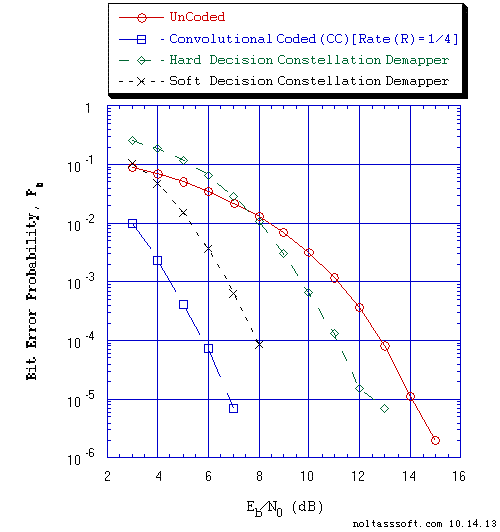Figure 10. Bit Error Probability for UnCoded, Rate = 1/4 Convolutional Coded, and
Rate Matching R = 2/4 Punctured Convolutional Coded (PCC) Non-Rectangular
(NR) 16-QAM Signaling over a Coherent Memoryless Channel with Additive
White Gaussian Noise (AWGN):
Parent Code Rate = 1/3, K = 4, (7, 5, 6, 7) a Non-Recursive Convolutional
Code and Viterbi Algorithm Decoder using a Path Memory Length of 48 bits
and a Hamming distance or a squared Euclidean distance Branch Metric;
Punctured Code (Rp = 2/4) derived from this 1/3 rate parent code for
Puncturing Period (P) of 2 using the P Matrix: [[1,0],[1,1],[0,1]]; &
PCC NR 16-QAM Demodulation Constellation Demapper Search Algorithms:
Search by Signal Vector Partitions (Hard Decision) or
Search by Bit Position and Value Partitions (Soft Decision).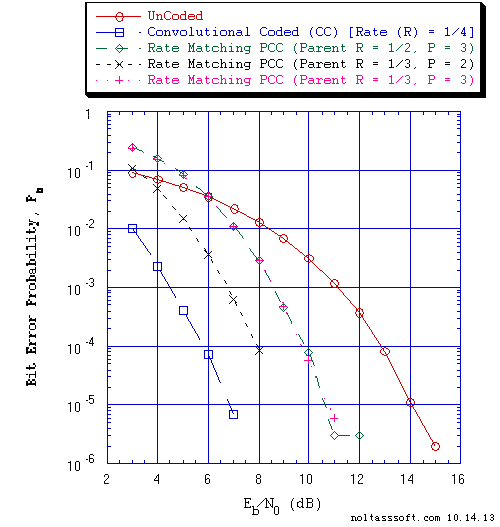Figure 11. Bit Error Probability for UnCoded, Convolutional Coded, and Rate
Matching Punctured Convolutional Coded  (PCC) Non-Rectangular (NR)
16-QAM Signaling over a Coherent Memoryless Channel with Additive
White Gaussian Noise (AWGN):
Rate = 1/4, Constraint Length K = 4,(15,9,7,15), Parent Code Rates = 1/2,
K = 4, (3,2,3,3), & 1/3, K = 4, (7,5,6,7) Best (Optimal) Non-Recursive
Convolutional Codes and Viterbi Algorithm Decoder using a Path Memory
Length of 48 bits and a squared Euclidean distance Branch Metric;
PCC Rate = 3/4 code derived from the 1/2 rate parent code for Puncturing
Period P = 3 using a P Matrix: [[1,0,0],[1,1,1]];
PCC Rate = 2/4 code derived from the 1/3 rate parent code for P = 2 using
a P Matrix [[1,0],[1,1],[0,1]]; &
PCC Rate = 3/4 code derived from the 1/3 rate parent code for P = 3 using
a P Matrix [[1,0,0],[1,1,0],[0,0,1]].
PCC NR 16-QAM Demodulation Constellation Demapper: Search by Bit
Position and Value Partitions (Soft Decision).
Note that the 16-QAM Non-Rectangular signal vector locations (constellation) are based on a modified square constellation such that each corner vector is moved diagonally by a counterclockwise rotation to the first available location next to the I or Q axis. And each signal vector's Bit-to-M-ary Channel Input Symbol assignment is based upon a Gray Code scheme.

Further, it is important to realize that one 16-QAM channel symbol is transmitted and received per a trellis branch for the Non-Rate Matching Convolutional Coded (CC) signaling cases. And for the Rate Matching (RM) Punctured Convolutional (PCC) signaling cases, one 16-QAM channel symbol is transmitted and received per a Punctured Period of trellis branches.

One must note that the Viterbi Algorithm (VA) decoding process for RM PCC Signaling that uses Soft Decision Demodulation per Puncturing Period (a multiple of trellis branches) is more complicated than the VA decoding process for Non-Rate Matching CC Signaling that uses a Soft Decision Unquantized Branch Metric per trellis branch. Its (RM PCC) Branch Metric uses Bit Metrics that are based on a squared Euclidean distance per bit per trellis branch. But in his 1992 paper, E. Zehavi (Reference No. 3, page 879) states that this Bit Metric (a suboptimal metric) does not satisfy the conditions for a distance metric.

Figure 10 focuses on the comparison of the two types of the Demapper Search algorithm for the use of a punctured code of rate Rp = 2/4 [Parent Code Rate = 1/3 with a Puncturing Period (P) of 2] and Non-Rectangular 16-QAM M-ary Signaling. Observe from Figure 10 BER plot the dramatic ~3.2 dB reduction in the required Eb/N0 dB to achieve the BER of 1.0e-4 that is due to the use of a Soft Decision SV Demapper as compared to the use of a Hard Decision SV Demapper.

Next, we look at the BER plot in Figure 11 as compared to the BER plot in the Figure 9 above (Section III) for all three punctured code rates [Rp = 3/4 (Parent Rate = 1/2, P = 3), Rp = 2/4 (Parent Rate = 1/3, P = 2), and Rp = 3/4 (Parent Rate = 1/3, P = 3)]. The simulated BER results that are displayed in Figure 9 plot are based on the use of a Hard Decision SV Demapper model in the simulations. One observes a dramatic reduction in Eb/N0 dB to achieve the BER of 1.0e-4 for all three punctured code rates Rp = 3/4, Rp = 2/4, and Rp = 3/4. This Eb/N0 dB reduction is due to the use of Bit Metrics that are produced by the Soft Decision Constellation Demapper.

It appears now that T1 Professional (T1 Version 2) has become a more valuable tool that is easy-to-use and very powerful given the addition of the Punctured Convolutional Code (PCC), Rate Matching (RM), and the RM PCC Soft Decision Constellation Demapper (Bit Metrics) capabilities to T1 that will complement/supplement T1 Professional's Non-Square M-ary QAM capability.

The Digital Communication System Designer can use T1 Version 2 for the purpose of selection of a particular Convolutional Code or Punctured Convolutional Code (High-Rate code) and signal set design for a bandwidth-constrained and power-constrained communication system such as today's next generation Wireless communication system.